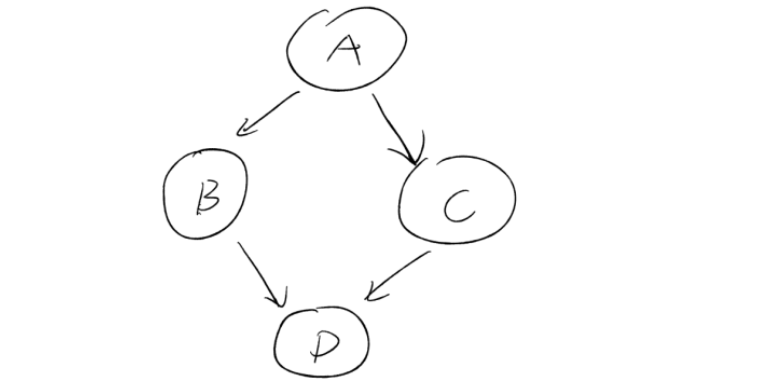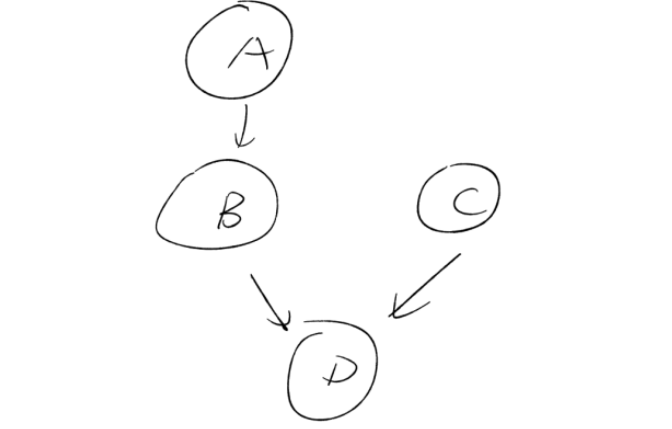# Python 经典面试题（一）

### 一、浮点数运算

#### 题目

 a = 0.1 b = 0.2 c = 0.3 assert a + b == c

#### 解决方案：

 from decimal import Decimal ​ a = 0.1 b = 0.2 c = 0.3 assert Decimal(str(a)) + Decimal(str(b)) == Decimal(str(c))

Decimal() 可以维持浮点数的精度，在金融领域和测试领域等精度要求高的行业有非常大的作用。 但是一定要注意： Decimal() 传的参数一定要是字符串类型，如果是数据类型会再次丢掉精度。

  Decimal(0.1) + Decimal(0.2) == Decimal(0.3)  # False   Decimal('0.1') + Decimal('0.2') == Decimal('0.3')  # True

### 二、列表的扁平化和降维

#### 题目

 groups = [['huahua', 'xiaojian'], ['musen', 'yuze'], ['keyou']] # 得到结果 ['huahua', 'xiaojian', 'musen', 'yuze', 'keyou']

#### 方法一

 names = [] for group in groups:     for name in group:         names.append(name) print(names)

#### 方法二

 names = sum(groups, [])

 a = sum([1,2,3])

 a = sum([1,2,3], 0) # 0 + 1 + 2 + 3

a = sum([['yuze'], ['is'], ['a']], [])
# [] + ['yuze'] + ['is'] + ['a']
# 列表拼接


#### 方法三

a = [e for group in groups for e in group]


### 三、多重继承

class A:
def run(self):
print("a is running")

class B:
def run(self):
print("b is running")

class C(A, B):
pass

# 会打印什么？？？
C().run()


#### 菱形问题

class A:
def run(self):
print("a running")

class B(A):
pass

class C(A):
def run(self):
print("C running")

class D(B, C):
pass

# 会打印什么？？？
D().run()class A:
def run(self):
print("a running")

class B(A):
pass

class C:
def run(self):
print("C running")

class D(B, C):
pass

# 会打印什么？？？
D().run()#### 题目解析

(<class '__main__.D'>, <class '__main__.B'>, <class '__main__.C'>, <class '__main__.A'>, <class 'object'>)


D 排在最前面，如果 D 定义了 run 方法，就会被优先使用，然后是 B, 然后是C， 然后是 A, 最后是 object 基类。

(<class '__main__.D'>, <class '__main__.B'>, <class '__main__.A'>, <class '__main__.C'>, <class 'object'>)


A 和 C 互换了顺序。造成 2 个题目的答案不一致。

=====请大家尊重原创，如要转载，请注明出处：转载自：https://www.cnblogs.com/wagyuze，且在文章页面明显位置给出原文连接，否则保留追究法律责任的权利。=====
• =====有任何疑问，欢迎加微信 qd20150815 (加时请备注：博客园-雨泽）=====

• posted @ 2019-10-12 14:33  王雨泽  阅读(2212)  评论(0编辑  收藏  举报